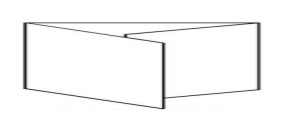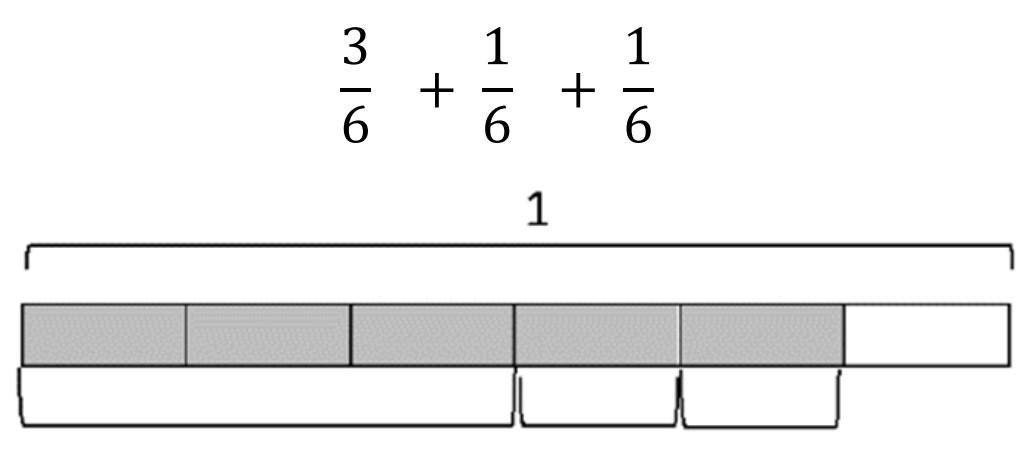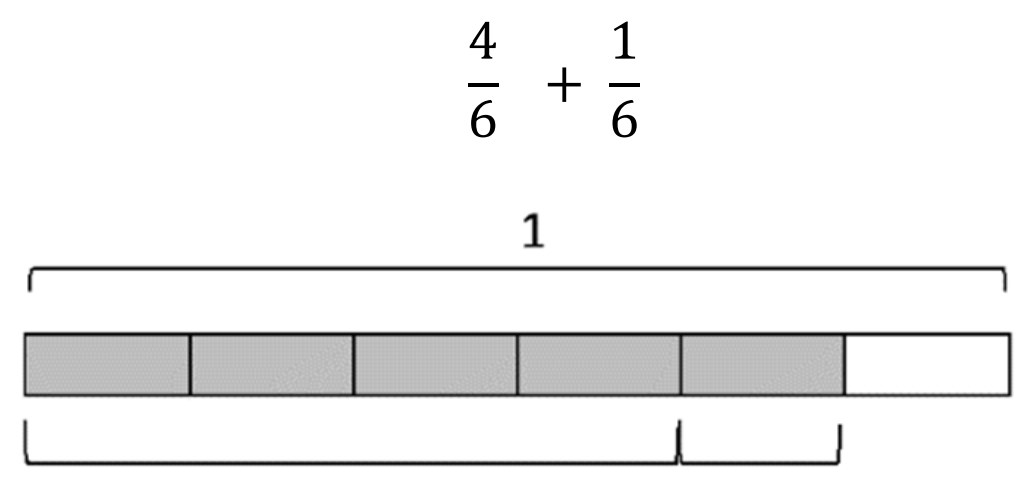# 4th Grade, Activity 237: Build Fractions from Unit Fractions by Applying and Extending Previous Understandings of Operations on Whole Numbers

Understand a fraction a/b with a > 1 as a sum of fractions 1/b. (Denominators are limited to 2, 3, 4, 5, 6, 8, 10, 12, and 100.)

a. Understand addition and subtraction of fractions as joining and separating parts referring to the same whole. (Whole Numbers)

Example: ¾ = ¼ + ¼ + ¼

### Modeling Fractions with Strip Diagrams

• Group: Whole Class/Alone
• Materials: adding machine strips, Modeling Fractions with Strip Diagrams sheet, map pencils

Issue a strip of adding machine tape and map pencils to each student. Direct students on how to fold the strip into sixths. Students first fold their strip into thirds as shown below. Once the paper is folded into thirds, fold it again to form sixths.After the paper is folded, instruct students to write 16 in each section. Write 56 on the board. Have students to lightly shade 56 of their strip with a map pencil.

Next, have students write the decomposition as a sum of unit fractions. (16 + 16 + 16 + 16 + 16). Then have them record the decomposition in a variety of other ways. See the examples below.Give students a Modeling Fractions with Strip Diagrams sheet. Instruct students to write a number sentence to match each diagram. Work through the first example with students. Reinforce students’ understanding of finding the sum of fractions.